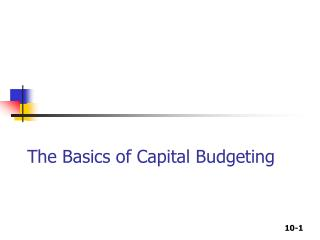DownloadDownload PresentationThe Basics of Capital Budgeting

The Basics of Capital Budgeting

Télécharger la présentationThe Basics of Capital Budgeting

- - - - - - - - - - - - - - - - - - - - - - - - - - - E N D - - - - - - - - - - - - - - - - - - - - - - - - - - -
Presentation Transcript

1. The Basics of Capital Budgeting

2. What is capital budgeting? • Analysis of potential additions to fixed assets. • Long-term decisions; involve large expenditures. • Very important to firm’s future.

3. What is the difference between independent and mutually exclusive projects? • Independent projects – if the cash flows of one are unaffected by the acceptance of the other. • Mutually exclusive projects – if the cash flows of one are adversely impacted by the acceptance of the other.

4. What is the difference between normal and nonnormal cash flow streams? • Normal cash flow stream – Cost (negative CF) followed by a series of positive cash inflows. One change of signs. • Nonnormal cash flow stream – Two or more changes of signs. Most common: Cost (negative CF), then string of positive CFs, then cost to close project.

5. Capital budgeting decision rules Five methods are used to evaluate projects: (1) Payback (2) Discounted payback (3) Net Present Value (NPV) (4) Internal Rate of Return (IRR) (5) Modified Internal Rate of Return (MIRR)

6. What is the payback period? • The number of years required to recover a project’s cost, or “How long does it take to get our money back?” • Calculated by adding project’s cash inflows to its cost until the cumulative cash flow for the project turns positive.

7. 2.4 3 0 1 2 Project L 80 CFt-100 10 60 100 Cumulative -100 -90 0 50 -30 30 80 PaybackL = 2 + / = 2.375 years = 1.6 3 0 1 2 Project S CFt-100 70 100 20 50 Cumulative -100 0 20 40 -30 30 50 PaybackS = 1 + / = 1.6 years = Calculating payback

8. Strengths and weaknesses of payback • Strengths • Provides an indication of a project’s risk and liquidity. • Easy to calculate and understand. • Weaknesses • Ignores the time value of money. • Ignores CFs occurring after the payback period.

9. 2.7 3 0 1 2 10% CFt -100 10 60 80 PV of CFt -100 9.09 49.59 60.11 Cumulative -100 -90.91 18.79 -41.32 Disc PaybackL = 2 + / = 2.7 years = 41.32 60.11 Discounted payback period • Uses discounted cash flows rather than raw CFs.

10. Net Present Value (NPV) • Sum of the PVs of all cash inflows and outflows of a project:

11. What is Project L’s NPV? YearCFtPV of CFt 0 -100 -\$100 1 10 9.09 2 60 49.59 3 80 60.11 NPVL = \$18.79 NPVS = \$19.98

12. Rationale for the NPV method NPV = PV of inflows – Cost = Net gain in wealth • If projects are independent, accept if the project NPV > 0. • If projects are mutually exclusive, accept projects with the highest positive NPV, those that add the most value. • For example, accept S if mutually exclusive (NPVs > NPVL), and would accept both if independent.

13. Internal Rate of Return (IRR) • IRR is the discount rate that forces PV of inflows equal to cost, and the NPV = 0: • Solving for IRR with a financial calculator: • Enter CFs in CFLO register. • Press IRR; IRRL = 18.13% and IRRS = 23.56%.

14. How is a project’s IRR similar to a bond’s YTM? • They are the same thing. • Think of a bond as a project. The YTM on the bond would be the IRR of the “bond” project. • EXAMPLE: Suppose a 10-year bond with a 9% annual coupon sells for \$1,134.20. • Solve for IRR = YTM = 7.08%, the annual return for this project/bond.

15. Rationale for the IRR method • If IRR > WACC, the project’s rate of return is greater than its costs. There is some return left over to boost stockholders’ returns.

16. IRR Acceptance Criteria • If IRR > k, accept project. • If IRR < k, reject project. • If projects are independent, accept both projects, as both IRR > k = 10%. • If projects are mutually exclusive, accept S, because IRRs > IRRL.

17. NPV Profiles • A graphical representation of project NPVs at various different costs of capital. k NPVLNPVS 0 \$50 \$40 5 33 29 10 19 20 15 7 12 20 (4) 5

18. Drawing NPV profiles NPV (\$) 60 . 50 . 40 . Crossover Point = 8.7% . 30 . . IRRL = 18.1% 20 . . S . IRRS = 23.6% 10 L . . Discount Rate (%) 0 5 15 20 23.6 10 -10

19. Comparing the NPV and IRR methods • If projects are independent, the two methods always lead to the same accept/reject decisions. • If projects are mutually exclusive … • If k > crossover point, the two methods lead to the same decision and there is no conflict. • If k < crossover point, the two methods lead to different accept/reject decisions.

20. 0 1 2 k = 10% -800 5,000 -5,000 Project P has cash flows (in 000s): CF0 = -\$800, CF1 = \$5,000, and CF2 = -\$5,000. Find Project P’s NPV and IRR. • Enter CFs into calculator CFLO register. • Enter I/YR = 10. • NPV = -\$386.78. • IRR = ERROR Why?